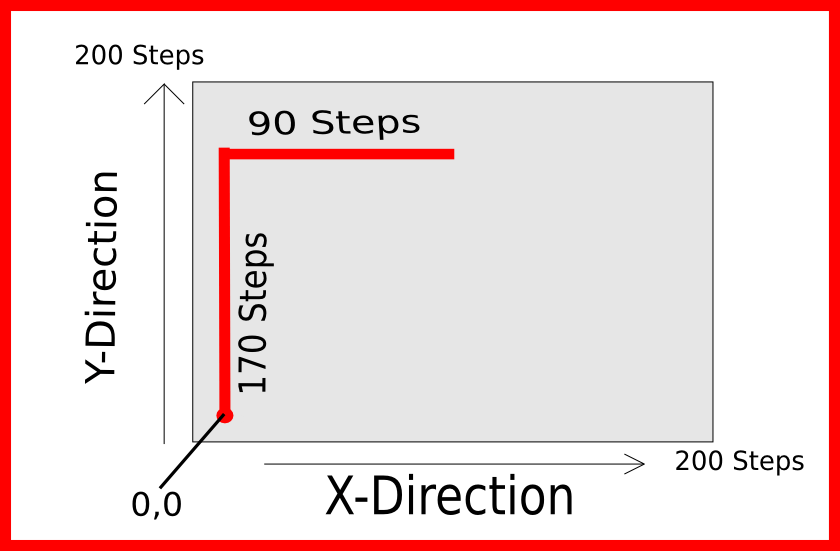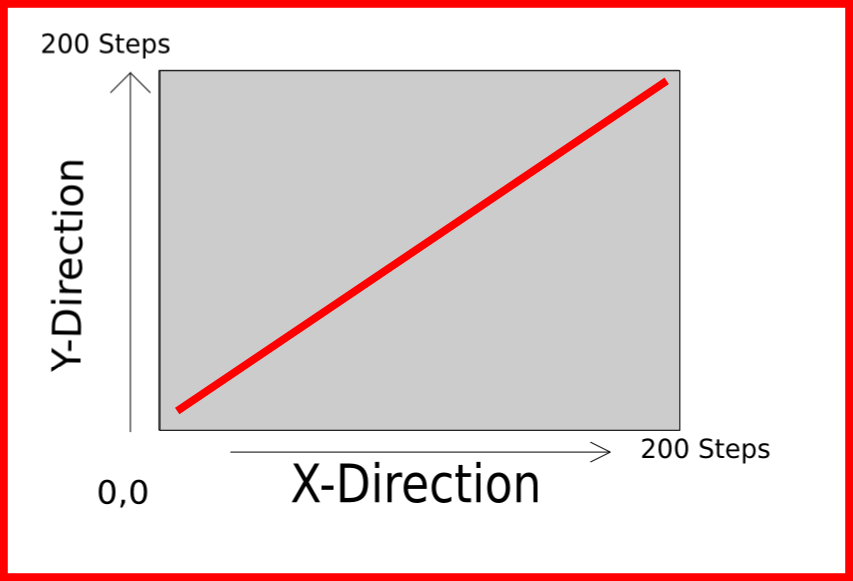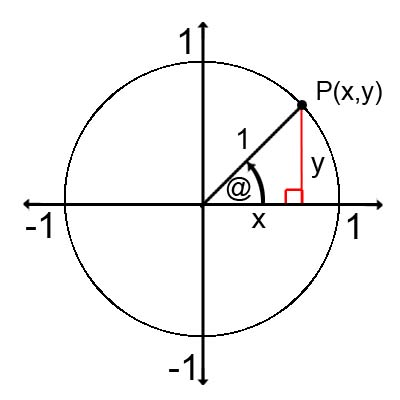# Second attempt at making an x-y plotter (TinyPlotter)

This plotter was built using parts from cd disc drives. The parts that actually control the motion are the steppers in the cd drives. The steppers are normally used to move a laser diode back and forth over the disc to read it. For this project, a separate disc drive was attached perpendicular to the laser part of the first drive. This allowed for an x-y movement by driving each of the steppers.

Here are some videos of it working:

Started off with squares:

Then went on to circles:

Then decided to really push the boat out and draw a circle in a square:

Theory of operation
At first glance something like this can seem quite complex. If the process is broken down into simple steps, it becomes apparent what exactly is going on.

The steps are:
1. Creating a function that control an individual stepper. This function accepts an whole number, representing the amount of steps required. I used four functions of this type, two for each direction of each stepper.

2. Creating a function that uses the stepper control functions to go to certain x-y points. This function accepts two numbers ( the x and y co-ordinates ).

3. Using equations to spit out these x-y co-ordinates and feed them into the previous function.

Starting at the beginning, we need a function that can accept a whole number and will then go and step the stepper motor as many times as it was asked in a certain direction. The gif below will serve as an example to show what these functions are actually doing. ( Source: http://commons.wikimedia.org/wiki/File:StepperMotor.gif ).

Two functions are required for each steppers, one for clockwise movement and one for anticlockwise. Just for easiness I will refer to clockwise movement as positive steps, and counter-clockwise as negative steps. It will become apparent why this is easier in a bit. There are two steppers, one for x-movement and one for y-movement and we need two functions for each, so lets call them:

x_steps_pos(int n)
x_steps_neg(int n)

y_steps_pos(int n)
y_steps_neg(int n)

Referring to the above gif of a stepper motor. If we wanted that to rotate clockwise for 8 steps, the c-code used would look like x_steps_pos(8); , then if you wanted it to go 3 steps back for example x_steps_neg(3); ( presuming that this is the x-direction stepper ), this could also be done for the y-direction stepper using the y_step functions.

Even with these basic function it would be possible to get things moving and drawing lines like the one in the image below.x-y plane

Using the stepper functions to draw a line like that would simply be:

```y_step_pos(170);
x_step_pos(90);
```

Presuming that the starting point was (0,0), after running both of those functions the marker would be left at point (90,170). Notice that its drawn half a rectangle, if you wanted to draw the other half you could just add in the following lines of code:

```y_step_neg(170);
x_step_neg(90);
```

and it would finish off the rectangle, leaving the marker where it started off at (0,0).

At this point it might be kind of difficult to see how this could draw a circle or any shape that isn’t a square or rectangle. The below example helps to see how something like a circle is achieved. if you wanted to draw a line from one corner to the other, at a 45 degree angle to the x and y axes, all that is required is the step the x motor once, then step the y motor once and repeat this all the way across .45 degree line plot

The c-code would look something like:

```while(n&lt;200)
{
x_step_pos(1);
y_step_pos(1);
n++;
}
```

This effectively just goes right one step, up one step, right one step, up one step, this process repeats until until 200 steps have been completed.

Now for step 2:

Making a function that can go from point to point. To do this some basic information is needed. Where am I now? ( what is my current x-y co-ordinate ), Where do I want to go to ( what x-y point do I want to get to). Then using a very simple formula (if you could even call it that), to work out how many steps you need for each motor to get to a certain x-y point simply subtract where you want to go to from where you are now. Its easier to look at some examples:

Lets say everything was just turned on, the marker is at point (0,0). The point we want to get to is say (100,150).

```//where i am now = (0,0)
//where i want to go to = (100,150)

//first work out how many steps are required on the x-axis:
//x_steps_required = (where I want to go to) - (where I am now)
x_steps_required = 100 - 0;
x_steps_required = 100;

//Then how many are required on the y-axis:
//y_steps_required = (where I want to go to) - (where I am now)
y_steps_required = 150 - 0;
y_steps_required = 150;
```

This tells us we need to 100 steps on the x-axis and 150 steps on the y-axis. The next step is to send these numbers to our stepper control function from earlier. The code would look like this:

```x_steps_pos(100);
y_steps_pos(150);
```

Now lets say you want to go from this point to another point, say for example the point (80,170). Going through the function again would look like this:

```//where i am now = (100,150)
//where i want to go to = (80,170)

//x_steps_required = (where I want to go to) - (where I am now)
x_steps_required = 80 - 100;
x_steps_required = -20;

//y_steps_required = (where I want to go to) - (where I am now)
y_steps_required = 170 - 140;
y_steps_required = 30 ;
```

This tells us we need 20 steps in the negative direction on the x-axis and 30 steps in the positive direction on the y-axis. Again these values would be passed to the stepper control functions. Notice that the negative 20 gets passed to the x_steps_neg function.

```x_steps_neg(20);
y_steps_pos(30);
```

In this project all of the code involved in calculating the x and y steps required to get to a point, and then sending those numbers to the stepper control functions was contained in a function called x_y_plot(int x, int y) . It accepts two arguments, the x and y co-ordinates.

At this point its fairly easy to start drawing more interesting shapes than squares and rectangles. A simple example of this would be drawing a circle. The first question is, how do we get a set of x-y points that represent the outline of a circle?. It turns out this is a very easy question solved with basic trigonometry.

This picture represents the idea fairly well apart from the ‘@’ symbol where the theta symbol should be.The code is then fairly simple:

```int theta = 0;
while(theta &lt; 360)
{

y = 100 +(r*sin(theta) ;
x = 100+(r*cos(theta) ;
x_y_plot(x,y);
theta++;
}

```

Here is the code I used for the TinyPlotter:

This entry was posted in C, Microcontrollers, pic microcontrollers, Plotters, Projects and tagged , , , , , , , . Bookmark the permalink.

### 2 Responses to Second attempt at making an x-y plotter (TinyPlotter)

1.batchloaf says:

Hi Shane,

I’m marking this one as your mini-project. I think this was an outstanding piece of work, so I’m giving it 9/10. It’s very impressive!

Ted

•shaneormonde says:

cool, thanks Ted.